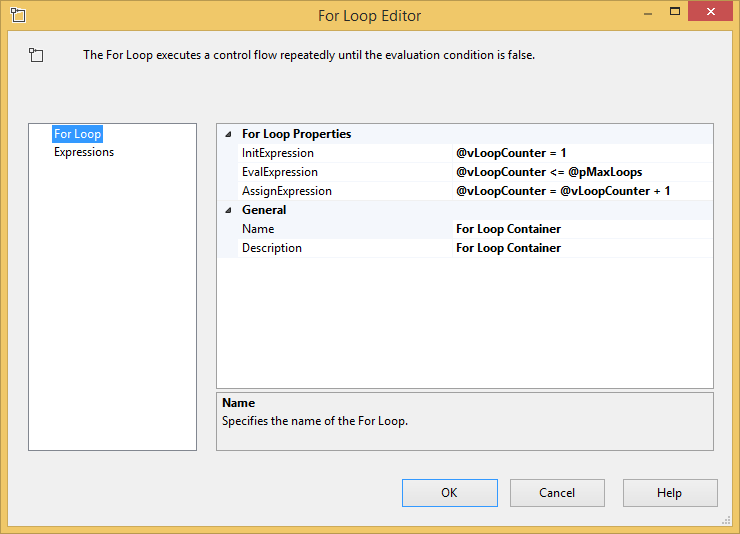# Get Started with the For Loop Container in SSIS

SQL Server Integration Services is equipped with tasks and containers to make it easy to design and maintain the flow of ETL: which logic should be executed, when should it be executed, and how many times should it occur. Most SSIS developers are familiar with the sequence container and the For Each Loop container, which can be used to group together tasks and execute the same logic a discrete number of times. In addition to these, there is a lesser-known but still very useful container for controlling logic flow: the For Loop container.

In this SSIS Basics post, I’ll briefly share how to use the For Loop container in SSIS.

### The For Loop Container in SSIS

Simply put, the For Loop container executes its ETL logic zero to n times. It has three functions that control how many times the loop will be executed:

• InitExpression: Used for the initial setting of a starting variable (such as setting a counter variable to zero).
• EvalExpression: This is the expression that evaluates whether the loop should continue. Of the three functions described here, this is the only one that requires a value – the others are optional.
• AssignExpression: This allows the use of an assignment expression, such as incrementing a loop counter.

For those with a programming background, this look very much like a for() loop statement in the C-derived languages. Functionally, it works in the exact same way as the for() loop, by continuing to execute the contained logic as long as the control condition remains true. This helps to draw contrast between the For Each Loop and the For Loop in SSIS. The former is list-based, and will execute for every item in the list supplied to it. The latter is value-based, and will execute as long as the EvalExpression is true.

In fairness, most ETL loads lean toward the list-based approach, but there are valid cases where a value-based approach is necessary. Some of those include:

• Processing a fixed subset of data
• Sampling for test or validation purposes
• Forcing a “wait state” until some milestone is reached
• Allowing the loop logic to be executed for some specified amount of time

### Configuring the For Loop Container

As noted above, the only value that is required for the For Loop container is the EvalExpression. A very simple For Loop configuration is shown below.The above supplies only the required value – a value of true to the EvalExpression. However, this is a very poorly configured For Loop, because the loop will continue executing indefinitely! True will always be true, so there is no logical end to this loop.

A more practical design pattern would use either an initialization expression, an assignment expression, or possibly both, to constrain the number of iterations. A simple example of this is shown below. I set up an SSIS package variable, typed as an Integer and named @vLoopCounter, with a default value of 0. In the For Loop settings, I’ve used the EvalExpression to check to see if this value is less than 10, and I use the AssignExpression to increment the @vLoopContainer value by 1 for every iteration of the loop.This example works, executing any logic contained in the For Loop exactly ten times. However, this pattern is very static. What if I want to increase the value expression to let the loop run more than 10 times? I’d need to open the package and modify it. Fortunately, the configurations expressions allow for the use of both variables and parameters. Below, the package has a couple of updates: an initial value of 1 is set for the @vLoopCounter variable, and the static comparison in EvalExpression is replaced by using the package parameter @pMaxLoops for the maximum number of loops.In the example above, the number of maximum loops can be specified at runtime, making for a more dynamic pattern.

The examples above show only an iteration based on the number of times the loop has run. Keep in mind when using the For Loop container, this logic can be based on any statement we choose: whether a particular set of files exist, how long the For Loop has been running, how many records have been processed, or some other custom metric specified in the expression. Even with a small number of inputs controlling how many times the For Loop container will execute, the possible uses for this are many, and can be as complex as needed.

### Conclusion

The For Loop container provides another way to execute repeating logic in SSIS. By using an approach similar to the for() loop in structured programming languages, the For Loop container adds more ETL flexibility through a value-based iterative pattern.